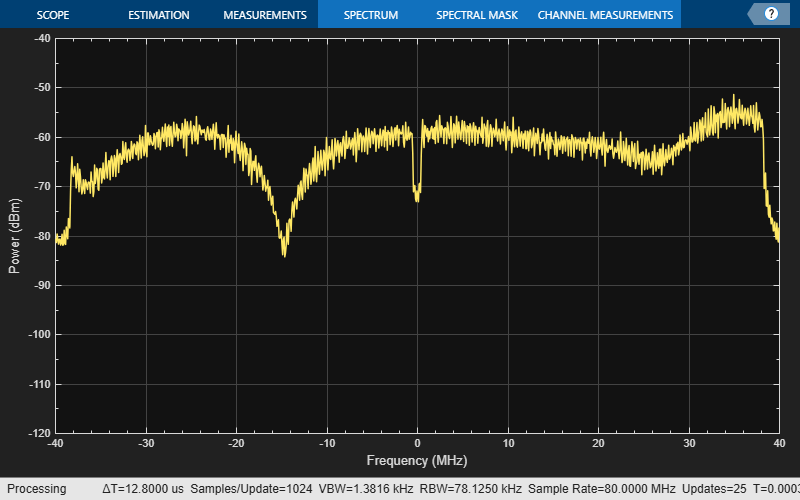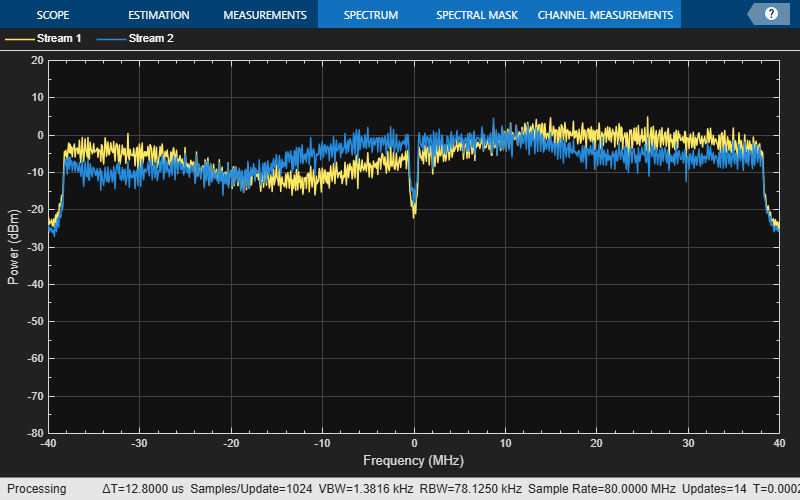# wlanTGacChannel

Filter signal through 802.11ac multipath fading channel

## Description

The `wlanTGacChannel` System object™ filters an input signal through an 802.11ac™ (TGac) multipath fading channel.

The fading processing assumes the same parameters for all NT-by-NR links of the TGac channel, where NT is the number of transmit antennas and NR is the number of receive antennas. Each link comprises all multipaths for that link.

To filter an input signal using a TGac multipath fading channel:

1. Create the `wlanTGacChannel` object and set its properties.

2. Call the object with arguments, as if it were a function.

## Creation

### Syntax

``tgac = wlanTGacChannel``
``tgac = wlanTGacChannel(Name,Value)``

### Description

example

````tgac = wlanTGacChannel` creates a TGac fading channel System object, `tgac`. This object filters a real or complex input signal through the TGac channel to obtain the channel-impaired signal.```
````tgac = wlanTGacChannel(Name,Value)` creates a TGac channel object, `tgac`, and sets properties using one or more name-value pairs. Enclose each property name in quotes. For example, `wlanTGacChannel('NumReceiveAntennas',2,'SampleRate',10e6)` creates a TGac channel with two receive antennas and a 10-MHz sample rate.```

## Properties

expand all

Unless otherwise indicated, properties are nontunable, which means you cannot change their values after calling the object. Objects lock when you call them, and the `release` function unlocks them.

If a property is tunable, you can change its value at any time.

Sample rate of the input signal in Hz, specified as a positive scalar.

Data Types: `double`

Delay profile model, specified as `'Model-A'`, `'Model-B'`, `'Model-C'`, `'Model-D'`, `'Model-E'`, or `'Model-F'`. To enable the `FluorescentEffect` property, select either `'Model-D'` or `'Model-E'`.

The table summarizes the models properties before the bandwidth reduction factor.

ParameterModel
ABCDEF
Breakpoint distance (m)555102030
Maximum delay (ns)0802003907301050
Rician K-factor (dB)000366
Number of taps1914181818
Number of clusters122346

The number of clusters represents the number of independently modeled propagation paths.

Data Types: `char` | `string`

Channel bandwidth, specified as `'CBW20'`, `'CBW40'`, `'CBW80'`, or `'CBW160'`. The default is `'CBW80'`, which corresponds to an 80 MHz channel bandwidth.

Data Types: `char` | `string`

RF carrier frequency in Hz, specified as a positive scalar.

Data Types: `double`

Speed of the scatterers in km/h, specified as a positive scalar.

Data Types: `double`

Distance between the transmitter and receiver in meters, specified as a positive scalar.

`TransmitReceiveDistance` is used to compute the path loss, and to determine whether the channel has a line of sight (LOS) or non line of sight (NLOS) condition. The path loss and standard deviation of shadow fading loss depend on the separation between the transmitter and the receiver.

Data Types: `double`

Normalize path gains, specified as a numeric or logical `1` (`true`) or `0` (`false`). To normalize the fading processes such that the total power of the path gains, averaged over time, is 0 dB, set this property to `1` (`true`). Otherwise, set this property to `0` (`false`).

Data Types: `logical`

User index, specified as a nonnegative integer. This property specifies the single user or a particular user in a multiuser scenario.

Data Types: `double`

Transmission direction of the active link, specified as either `'Downlink'` or `'Uplink'`.

Data Types: `char` | `string`

Number of transmit antennas, specified as a positive integer.

Data Types: `double`

Distance between transmit antenna elements, specified as a positive scalar expressed in wavelengths.

`TransmitAntennaSpacing` supports uniform linear arrays only.

#### Dependencies

To enable this property, set the `NumTransmitAntennas` property to a value greater than `1`.

Data Types: `double`

Number of receive antennas, specified as a positive integer.

Data Types: `double`

Distance between receive antenna elements, specified as a positive scalar expressed in wavelengths.

`ReceiveAntennaSpacing` supports uniform linear arrays only.

#### Dependencies

To enable this property, set the `NumReceiveAntennas` property to a value greater than `1`.

Data Types: `double`

Large-scale fading effects applied in the channel, specified as `'None'`, `'Pathloss'`, `'Shadowing'`, or `'Pathloss and shadowing'`.

Data Types: `char` | `string`

Fluorescent effect, specified as a numeric or logical `1` (`true`) or `0` (`false`). To include Doppler effects from fluorescent lighting, set this property to `1` (`true`).

#### Dependencies

To enable this property, set the `DelayProfile` property to `'Model-D'` or `'Model-E'`.

Data Types: `logical`

Power line frequency in Hz, specified as `'50Hz'` or `'60Hz'`.

The power line frequency is 60 Hz in the United States and 50 Hz in Europe.

#### Dependencies

To enable this property, set the `FluorescentEffect` property to `1` (`true`) and the `DelayProfile` property to `'Model-D'` or `'Model-E'`.

Data Types: `char` | `string`

Normalize channel outputs by the number of receive antennas, specified as a numeric or logical `1` (`true`) or `0` (`false`).

Data Types: `logical`

Enable channel filtering, specified as a numeric or logical `1` (`true`) or `0` (`false`). To enable channel filtering, set this property to `1` (`true`). To disable channel filtering, set this property to `0` (`false`).

### Note

If you set this property to `0` (`false`), the `step` object function does not accept an input signal. In this case, the `NumSamples` and `SampleRate` properties determine the duration of the fading process realization.

Data Types: `logical`

Number of time-domain samples used to get path gain samples, specified as a positive integer.

#### Dependencies

To enable this property, set the `ChannelFiltering` property to `0` (`false`).

Data Types: `double`

Data type of impaired signal, specified as one of these values:

• `'double'` – Return the `pathGains` output as a double-precision matrix

• `'single'` – Return the `pathGains` output as a single-precision matrix

#### Dependencies

To enable this property, set the `ChannelFiltering` property to `0` (`false`).

Data Types: `char` | `string`

Source of random number stream, specified as `'Global stream'` or `'mt19937ar with seed'`.

If you set `RandomStream` to `'Global stream'`, the current global random number stream generates normally distributed random numbers. In this case, the `reset` function resets the filters only.

If you set `RandomStream` to ```'mt19937ar with seed'```, the mt19937ar algorithm generates normally distributed random numbers. In this case, the `reset` function also reinitializes the random number stream to the value of the `Seed` property.

Data Types: `char` | `string`

Initial seed of an mt19937ar random number stream, specified as a nonnegative integer. The `Seed` property reinitializes the mt19937ar random number stream in the `reset` function.

#### Dependencies

To enable this property, set the `RandomStream` property to `'mt19937ar with seed'`.

Data Types: `double`

Enable path gain output computation, specified as a numeric or logical `1` (`true`) or `0` (`false`).

Data Types: `logical`

## Usage

### Syntax

``y = tgac(x)``
``[y,pathGains] = tgac(x)``
``pathGains = tgac(x)``

### Description

example

````y = tgac(x)` filters input signal `x` through the TGac fading channel defined by the `wlanTGacChannel` System object, `tgac`, and returns the result in `y`.```
````[y,pathGains] = tgac(x)` also returns in `pathGains` the TGac channel path gains of the underlying fading process. This syntax applies when you set the `PathGainsOutputPort` property to `1` (`true`).```
````pathGains = tgac(x)` returns the path gains. The `NumSamples` property determines the duration of the fading process.This syntax applies when you set the `ChannelFiltering` property to `0` (`false`).```

### Input Arguments

expand all

Input signal, specified as a real or complex NS-by-NT matrix, where:

• NS is the number of samples.

• NT is the number of transmit antennas and must be equal to the `NumTransmitAntennas` property value.

Data Types: `single` | `double`
Complex Number Support: Yes

### Output Arguments

expand all

Output signal, returned as an NS-by-NR complex matrix, where:

• NS is the number of samples.

• NR is the number of receive antennas and is equal to the `NumReceiveAntennas` property value.

Data Types: `single` | `double`

Path gains of the fading process, returned as an NS-by-NP-by-NT-by-NR complex array, where:

• NS is the number of samples.

• NP is the number of resolvable paths, that is, the number of paths defined for the case specified by the `DelayProfile` property.

• NT is the number of transmit antennas and is equal to the `NumTransmitAntennas` property value.

• NR is the number of receive antennas and is equal to the `NumReceiveAntennas` property value.

Data Types: `single` | `double`

## Object Functions

To use an object function, specify the System object as the first input argument. For example, to release system resources of a System object named `obj`, use this syntax:

`release(obj)`

expand all

 `info` Characteristic information about TGn, TGah, TGac, and TGax multipath fading channels
 `step` Run System object algorithm `release` Release resources and allow changes to System object property values and input characteristics `reset` Reset internal states of System object

### Note

`reset`: If the `RandomStream` property of the System object is set to `'Global stream'`, the `reset` function resets the filters only. If you set `RandomStream` to `'mt19937ar with seed'`, the `reset` function also reinitializes the random number stream to the value of the `Seed` property.

## Examples

collapse all

Generate a VHT waveform and pass it through a TGac SISO channel. Display the spectrum of the resultant signal.

Set the channel bandwidth and the corresponding sample rate.

```bw = 'CBW80'; fs = 80e6; ```

Generate a VHT waveform.

```cfg = wlanVHTConfig; txSig = wlanWaveformGenerator(randi([0 1],1000,1),cfg); ```

Create a TGac SISO channel with path loss and shadowing enabled.

```tgacChan = wlanTGacChannel('SampleRate',fs,'ChannelBandwidth',bw, ... 'LargeScaleFadingEffect','Pathloss and shadowing'); ```

Pass the VHT waveform through the channel.

```rxSig = tgacChan(txSig); ```

Plot the spectrum of the received waveform.

```saScope = dsp.SpectrumAnalyzer('SampleRate',fs,'YLimits',[-120 -40]); saScope(rxSig) ```Because path loss and shadowing are enabled, the mean received power across the spectrum is approximately -60 dBm.

Create a VHT waveform having four transmit antennas and two space-time streams.

```cfg = wlanVHTConfig('NumTransmitAntennas',4,'NumSpaceTimeStreams',2, ... 'SpatialMapping','Fourier'); txSig = wlanWaveformGenerator([1;0;0;1],cfg); ```

Create a 4x2 MIMO TGac channel and disable large-scale fading effects.

```tgacChan = wlanTGacChannel('SampleRate',80e6,'ChannelBandwidth','CBW80', ... 'NumTransmitAntennas',4,'NumReceiveAntennas',2, ... 'LargeScaleFadingEffect','None'); ```

Pass the transmit waveform through the channel.

```rxSig = tgacChan(txSig); ```

Display the spectrum of the two received space-time streams.

```saScope = dsp.SpectrumAnalyzer('SampleRate',80e6, ... 'ShowLegend',true, ... 'ChannelNames',{'Stream 1','Stream 2'}); saScope(rxSig) ```Transmit a VHT-LTF and a VHT data field through a noisy 2x2 MIMO channel. Demodulate the received VHT-LTF to estimate the channel coefficients. Recover the VHT data and determine the number of bit errors.

Set the channel bandwidth and corresponding sample rate.

```bw = 'CBW160'; fs = 160e6;```

Create VHT-LTF and VHT data fields having two transmit antennas and two space-time streams.

```cfg = wlanVHTConfig('ChannelBandwidth',bw, ... 'NumTransmitAntennas',2,'NumSpaceTimeStreams',2); txPSDU = randi([0 1],8*cfg.PSDULength,1); txLTF = wlanVHTLTF(cfg); txDataSig = wlanVHTData(txPSDU,cfg);```

Create a 2x2 MIMO TGac channel.

```tgacChan = wlanTGacChannel('SampleRate',fs,'ChannelBandwidth',bw, ... 'NumTransmitAntennas',2,'NumReceiveAntennas',2);```

Create an AWGN channel noise, setting SNR = 15 dB.

```chNoise = comm.AWGNChannel('NoiseMethod','Signal to noise ratio (SNR)',... 'SNR',15);```

Pass the signals through the TGac channel and noise models.

```rxLTF = chNoise(tgacChan(txLTF)); rxDataSig = chNoise(tgacChan(txDataSig));```

Create an AWGN channel for a 160 MHz channel with a 9 dB noise figure. The noise variance, `nVar`, is equal to kTBF, where k is Boltzmann's constant, T is the ambient temperature of 290 K, B is the bandwidth (sample rate), and F is the receiver noise figure.

```nVar = 10^((-228.6 + 10*log10(290) + 10*log10(fs) + 9)/10); rxNoise = comm.AWGNChannel('NoiseMethod','Variance','Variance',nVar);```

Pass the signals through the receiver noise model.

```rxLTF = rxNoise(rxLTF); rxDataSig = rxNoise(rxDataSig);```

Demodulate the VHT-LTF. Use the demodulated signal to estimate the channel coefficients.

```dLTF = wlanVHTLTFDemodulate(rxLTF,cfg); chEst = wlanVHTLTFChannelEstimate(dLTF,cfg);```

Recover the data and determine the number of bit errors.

```rxPSDU = wlanVHTDataRecover(rxDataSig,chEst,nVar,cfg); numErr = biterr(txPSDU,rxPSDU)```
```numErr = 0 ```

## Algorithms

expand all

The algorithms used to model the TGac channel are based on those used for the TGn channel and are described in `wlanTGnChannel` and . The changes to support the TGac channel include:

• increased bandwidth

• higher-order MIMO

• multi-user MIMO

• reduced Doppler

Complete information on the changes required to support TGac channels can be found in .

 Erceg, V., L. Schumacher, P. Kyritsi, et al. TGn Channel Models. Version 4. IEEE 802.11-03/940r4, May 2004.

 Breit, G., H. Sampath, S. Vermani, et al.TGac Channel Model Addendum. Version 12. IEEE 802.11-09/0308r12, March 2010.

 Kermoal, J. P., L. Schumacher, K. I. Pedersen, P. E. Mogensen, and F. Frederiksen. “A Stochastic MIMO Radio Channel Model with Experimental Validation”. IEEE Journal on Selected Areas in Communications. Vol. 20, No. 6, August 2002, pp. 1211–1226.

##### SupportGet trial now